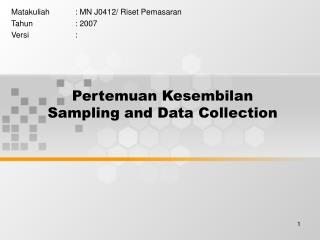DownloadDownload PresentationPertemuan Kesembilan Sampling and Data Collection

# Pertemuan Kesembilan Sampling and Data Collection

Download Presentation## Pertemuan Kesembilan Sampling and Data Collection

- - - - - - - - - - - - - - - - - - - - - - - - - - - E N D - - - - - - - - - - - - - - - - - - - - - - - - - - -
##### Presentation Transcript

1. Pertemuan KesembilanSampling and Data Collection Matakuliah : MN J0412/ Riset Pemasaran Tahun : 2007 Versi :

2. Learning Outcomes Pada akhir pertemuan ini, diharapkan mahasiswa akan mampu : • Mahasiswa dapat menyisihkan sampel dan populasi tanpa menghilangkan unsur

3. Outline Materi • Materi 1: Required Steps in Sampling • Materi 2: Types of Sampling Plans • Materi 3: Non Probability Samples • Materi 4:Probability Samples • Materi 5: Sample Size

4. 6-Step Procedure for Drawing a Sample Define the Target Population Step 1 Identify the Sampling Frame Step 2 Select a Sampling Procedure Step 3 Determine the Sample Size Step 4 Select the Sample Elements Step 5 Collect the Data from the Designated Elements Step 6

5. Classification of Sampling Techniques Sampling Designs • Nonprobability Samples • Convenience • Judgment • Quota • Probability Samples • Simple Random • Statified • Proportionate • Disproportionate • Cluster • Systematic • Area

6. n = 2 n = 5 n = 30 Distribution of Sample Means for Different Samples Sizes, Population Shapes Populations Sampling Distributions of mean

7. Relationship Between Parameters of Parent Population and Derived Population Parent Population Element Total A B C D E F 3 6 9 12 15 18 3 + 6 + 9 + 12 + 15 + 18  Xi N = = = 10.5 Mean:  6 Variance: 2  (Xi-)2 (3-10.5)2 + ... +(18-10.5)2 N = = 6 = 157.5 = 26.25 6

8. Mean Xj Derived Population of All Possible Distinguishable Samples Element Total 1 2 3 4 5 6 7 8 9 10 11 12 13 14 15 16 17 18 19 20 ABC ABD ABE ABF ACD ACE ACF ADE ADF AEF BCD BCE BCF BDE BDF BEF CDE CDF CEF DEF 18 21 24 27 24 27 30 30 33 36 27 30 33 33 36 39 36 39 42 45 6 7 8 9 8 9 10 10 11 12 9 10 11 11 12 13 12 13 14 15

9. =(6 -10.5)2 + (7-10.5)2 + ... + (15-10.5)2 20 Derived Population L  xj j =1 L Mean: 6 + 7 + ... + 15 105 E( x ) x = 10.5 = = = 20 20 L  ( xj j =1 L - E(x))2 Variance: 2 = _ x = 5.25

10. Distribution of Variables in Parent and Derived Population 1 Absolute Frequency 0 Values of X 3 2 Absolute Frequency 1 0 Values of x

11. n1 = 100  Xi1 x1 = = 3.2 n1  (Xi1 x1 )2 ^ s12 = = .14 n1 -1 n3 = 100  Xi3 x3 = = 5.8 n3  (Xi3 x3 )2 ^ s32 = = .20 n3 -1 Example Calculations of Generating a Confidence Interval with a Stratified Sample I II n2 = 100  Xi2 x2 = = 4.6 n2  (Xi2 x2 )2 ^ s22 = = .12 n2 -1 III IV n4 = 100  Xi4 x4 = = 7.2 n4  (Xi4 x4 )2 ^ s42 = = .18 n4 -1

12. L  L  h=1 h=1 2 Nh L L   sxst 2 N h=1 h=1 sxst Size and Strata in Parent Population I. N1 = 5000 25000 15000 5000 II. N2 = N = 50000 III. N3 = IV. N4 = Nhxh Whxh xst = = N = 1/10(3.2) + 5/10(4.6) + 3/10(5.8) + 1/10(7.2) = 5.08 ( ) ^ (sh)2 Whs2xh = = nh = (1/10)2 (.14) + (5/10)2 (.12) + (3/10)2 (.20) + (1/10)2 (.18) = .000512 100 100 100 100 =.0226